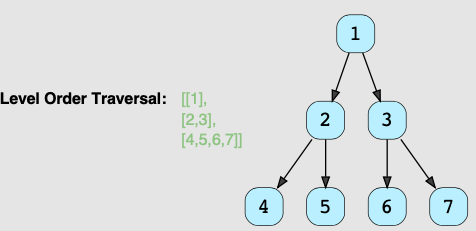Shawn Lin

2022-07-24

# BFS Traversal

Breadth first search, or BFS, is a common search algorithm for binary trees. In the question statement, when you see level order traversal, you should think about BFS immediately.

## List Tree Element Level by Level

When doing BFS, there are a few common patterns. They can all be pretty well summarized in the solution for the following question:

Given a binary tree, populate an array to represent level-by-level traversal. You should populate the values of all nodes of each level from left to right in separate sub-arrays.from collections import deque

def traverse(root):
result = []
queue = deque()

if root:
queue.append(root)

while queue:
size = len(queue)
level = []

for _ in range(size):
node = queue.popleft()
level.append(node.val)
if node.left:
queue.append(node.left)
if node.right:
queue.append(node.right)

result.append(level)

return result